C H A P T E R  5
 Working With Matrices

Most matrices can be stored in ways that save both storage space and computation time. Sun Performance Library uses the following storage schemes:

• Banded storage
• Packed storage

The Sun Performance Library processes matrices that are in one of four forms:

• General
• Triangular
• Symmetric
• Tridiagonal

Storage schemes and matrix types are described in the following sections.

## 5.1 Matrix Storage Schemes

Some Sun Performance Library routines that work with arrays stored normally have corresponding routines that take advantage of these special storage forms. For example, DGBMV will form the product of a general matrix in banded storage and a vector, and DTPMV will form the product of a triangular matrix in packed storage and a vector.

### 5.1.1 Banded Storage

A banded matrix is stored so the jth column of the matrix corresponds to the jth column of the Fortran array.

The following code copies a banded general matrix in a general array into banded storage mode.

 ``` C Copy the matrix A from the array AG to the array AB. The C matrix is stored in general storage mode in AG and it will C be stored in banded storage mode in AB. The code to copy C from general to banded storage mode is taken from the C comment block in the original DGBFA by Cleve Moler. C NSUB = 1 NSUPER = 2 NDIAG = NSUB + 1 + NSUPER DO ICOL = 1, N I1 = MAX0 (1, ICOL - NSUPER) I2 = MIN0 (N, ICOL + NSUB) DO IROW = I1, I2 IROWB = IROW - ICOL + NDIAG AB(IROWB,ICOL) = AG(IROW,ICOL) END DO END DO ```

This method of storing banded matrices is compatible with the storage method used by LAPACK and BLAS.

### 5.1.2 Packed Storage

A packed vector is an alternate representation for a triangular, symmetric, or Hermitian matrix. An array is packed into a vector by storing the elements sequentially column by column into the vector. Space for the diagonal elements is always reserved, even if the values of the diagonal elements are known, such as in a unit diagonal matrix.

An upper triangular matrix or a symmetric matrix whose upper triangle is stored in general storage in the array A, can be transferred to packed storage in the array AP as shown below. This code comes from the comment block of the LAPACK routine DTPTRI.

 ``` JC = 1 DO J = 1, N DO I = 1, J AP(JC+I-1) = A(I,J) END DO JC = JC + J END DO ```

Similarly, a lower triangular matrix or a symmetric matrix whose lower triangle is stored in general storage in the array A, can be transferred to packed storage in the array AP as shown below:

 ``` JC = 1 DO J = 1, N DO I = J, N AP(JC+I-1) = A(I,J) END DO JC = JC + N - J + 1 END DO ```

## 5.2 Matrix Types

The general matrix is the most common type, and most operations in the Sun Performance Library operate on the general matrix. In many cases, there are routines that will work with the other types of matrices. For example, DGEMM computes the product of two general matrices, and DTRMM computes the product of a triangular matrix and a general matrix.

### 5.2.1 General Matrices

The storage of a general matrix is such that there is a one-to-one correspondence between the elements of the matrix and the elements of the array. Element Aij of matrix A is stored in element A(I,J) of the corresponding array A. The general matrix has no special storage scheme since each of its elements is stored explicitly. In contrast, only the nonzero upper-diagonal, diagonal, and lower-diagonal elements of a general band matrix are stored. The following example shows how a general band matrix is stored in a two-dimensional array. Array locations marked with x are not accessed.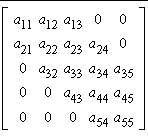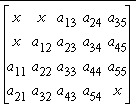General Band Matrix General Band Matrix in Packed Storage

### 5.2.2 Triangular Matrices

There are two storage schemes for a triangular matrix. In the unpacked scheme where the matrix is stored in a two-dimensional array, there is a one-to-one correspondence between all elements of the matrix and the elements of the array, but zero entries in the matrix are neither set nor accessed in the array (denoted by x). In the packed storage scheme, nonzero elements of the matrix are packed by column in a one-dimensional array.

A triangular matrix can be stored using packed storage.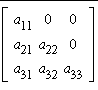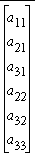Triangular Band Matrix Triangular Matrix in Unpacked Storage Triangular Matrix in Packed Storage

A triangular band matrix can be stored in packed storage using a two-dimensional array as shown below. Elements marked with x are not accessed..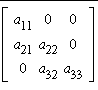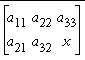Triangular Band Matrix Triangular Band Matrix in Packed Storage

### 5.2.3 Symmetric Matrices

A real symmetric or complex Hermitian matrix is similar to a triangular matrix in that only elements in its upper or lower triangle is explicitly stored in the corresponding elements of a two-dimensional array. The remaining elements of the array (denoted by x below) are neither set nor accessed. The active upper or lower triangle can also be packed by column into a one-dimensional array.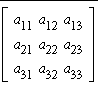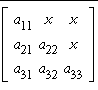Symmetric Matrix Symmetric Matrix in Unpacked Storage Symmetric Matrix in Packed Storage

### 5.2.4 Tridiagonal Matrices

A tridiagonal matrix has nonzero elements only on the main diagonal, the first superdiagonal, and the first subdiagonal. It is stored using three one-dimensional arrays.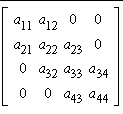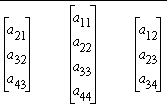Tridiagonal Matrix Storage for Tridiagonal Matrix